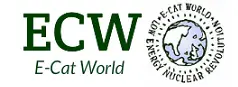# E-Cat QX Presentation Thread #3 — Full Video of Event, Comments from Mats Lewan, Measurements By Eng. William Hurley

The following comments have been sent to me from Mats Lewan. Since the film of the presentation is yet to be produced, this helps provide us with some more details of what was measured today.

‘I think the demonstration today went well, with some limits that depends on what Rossi will accept to measure publicly. The problematic part is that the voltage over the reactor could not be measured, which would be necessary to calculate the electric power consumed by the reactor. In the calculations made by Rossi and Eng. William S. Hurley, who oversaw the measurements, the power consumed by the 1-ohm resistor was used as input power instead, assuming that the plasma inside the reactor has a resistance close to that of a conductor, thus consuming a negligible amount of power since the voltage across the reactor would be very low.

The dummy measurements that I insisted to do, which can be seen in the slides, consisted in replacing the reactor, first with a conductor, then with a 800-ohm resistance. Using a conductor gave a similar electric situation as when the reactor was running—a voltage across the 1-ohm resistor of about 0.4V, slightly higher than the 0.3V measured with the reactor in the circuit. Using a 800-ohm resistor instead of the reactor the voltage across the 1-ohm resistor was about 20mV. At that point we also measured the total voltage over both the 1-ohm and the 800-ohm resistance together, basically the output voltage of the black box power supply, which was then about 12V. That is consistent with the 20mV voltage across the 1-ohm resistor (it should be 15mV).

The 800-ohm dummy was used since at the current flowing through the circuit with the reactor – about 0.3A – the reactor would have consumed 72W electric power if it had a resistance of 800ohms. However, this would have meant that that the voltage across the reactor would have been about 240V. The dummy measurement with the 800-ohm resistor indicated three things: 1. The voltage from the power supply only reached only about 12V as a maximum. 2. If the reactor had a resistance of that order of magnitude it would have resulted in a much lower current, about 20mA, than the one measured with the reactor in place. 3. The power consumed by such a resistance in the circuit would only be about 0.3W.

From the two dummy measurements we can also conclude that the black box power supply adapts its output according to the resistance in the circuit, or rather, to how the reactor behaves. The dummy resistance that most closely replicated the situation with the reactor was using a conductor, indicating that the reactor really behaves as a conductor. This means that the power consumed by the resistance and the reactor together was about 0.3 x 0.3 = 0.09W, as used in the calculations, but that in fact, the reactor consumed a negligible part of that power, resulting in a COP that could be in the order of tens of thousands or more.

We can make another possible conclusion. Since the voltage across the 1-ohm resistance, while using a conductor as a dummy, was about 0.4V, the total output voltage from the power supply at normal operation could be about 0.4V, indicating that the voltage across the reactor could be at the order of magnitude of 0.1V, and the power consumed at 0.3A would be about 0.03W. This would result in a COP of about 1,000. (I don’t remember the value of thermal output power from the reactor, as calculated by William Hurley, but I think ot was about 30W).

Having said this, it seems strange that the power supply, even if it is a complex design, is such that it needs significant active cooling, resulting in a total system that has a COP of about 1 or less at this point. On the other hand, this is what Rossi explained to be one of the challenges in further development of the system.’

UPDATE:

Mats also sent me the following:

DATA REPORT OF THE MEASUREMENTS MADE ON NOVEMBER 24TH 2017 ON THE E-CAT QUARKX TESTED AT THE IVA, GREV TUREGATAN 16, STOCKOLM, SWEDEN.

Duration of the measurement period: 1 hour: the measurement has been made after the apparatus has reached a reasonably constant temperature
amount of water pumped through the reactor: 1 000 g
Water temperature at the input of the reactor: 21 C
Water temperature at the output of the reactor: 41 C
Delta T: 20 C
Energy produced: 20 x 1.14 = 22.8 Wh/h
Measurement of the energy consumed ( during the hour for 30′ no energy has been supplied to the E-Cat) :
V: 0.3
OHM: 1
A: 0.3
Wh/h 0.09/2= 0.045
Ratio between Energy Produced and energy consumed: 22.8/0.045 = 506.66

Instrumentation used for the measurements:
Oscilloscope Tektronix TBS 1052B
K probes Omega supplied and calibrated by Prof. Bo Hoistad of the University of Uppsala
Water pump Prominent. The water pumped for 1 hour has been poured in a plastic container seat on a scale to measure exactly the water passed through the E-Cat.
Temperature Data Logger: PICO Technology
The scale to weight the water passed through the E-Cat has been supplied by Eng. Mats Lewan of Stockolm

William S. Hurley
Senior Engineer- Endeavor
Los Angeles